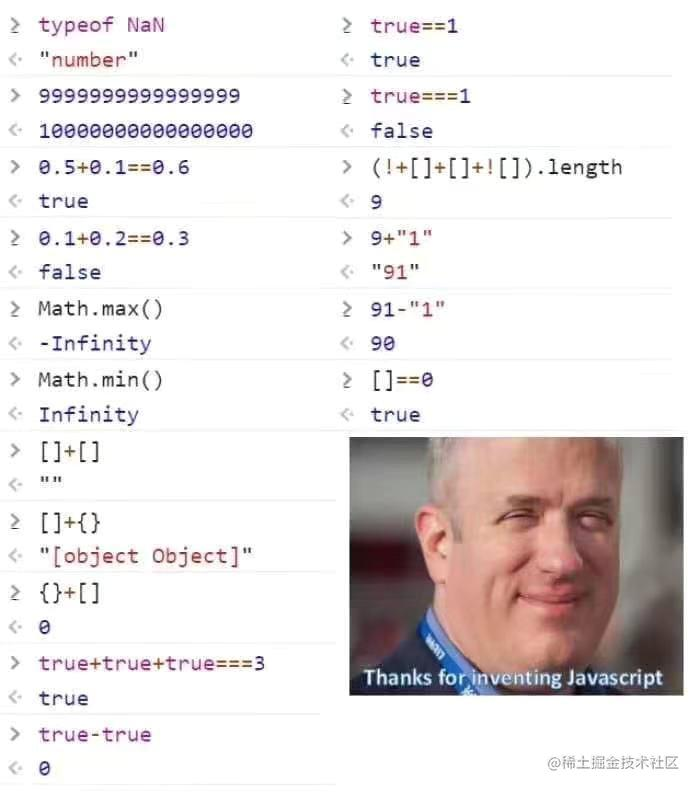09 July 2022

JavaScript 是一个动态类型语言，在运行时获取变量类型是常用操作，由于 JavaScript 设计的问题，看似简单的问题，在 JavaScript 中可能并不简单，比如在社区中流传的下图，仔细看一下这些坑，即便是 JavaScript 老司机也经常翻车。``````function double(x) {
// 0会被错误计算
if (!x) {
return NaN;
}
return x * 2;
}
``````

``````if (x === null || x === undefined) {
return NaN;
}
``````

``````window.undefined = 1;

// 判断不能生效
if (x === undefined) {
console.log(111);
}
``````

``````(function () {
var undefined = 1;
// 判断不能生效
if (x === undefined) {
console.log(111);
}
})();
``````

``````// null 和 undefined都会判断
if (x == null) {
}
``````

``````if (x === null || typeof x === 'undefined') {
}
``````

``````const x = Math.sqrt(-1); // NaN

// 注意这里的isNaN判断
if (typeof x === 'number' && !isNaN(x)) {
console.log(x.toFixed(2));
}
``````

``````Number.isNaN = function (value) {
return typeof value === 'number' && isNaN(value);
};
``````

typeof 只能判断基本类型，对于引用类型的到的值都是`object`

``````typeof []; // 'object'
typeof {}; // 'object'
typeof c; // 'object'
``````

`instanceof` 可以用来检测引用类型，其原理是检测 `constructor.prototype` 是否存在于参数 object 的原型链上

``````{} instanceof Object // true
[] instanceof Array // true
/reg/ instanceof RegExp // true
``````

instanceof 存在的一个问题是不够准确，原型链上存在的都会返回 true

``````[] instanceof Array // true
[] instanceof Object // true 注意这里
``````

``````function type(x) {
if (x instanceof Object) {
return 'object';
}

// Array永远得不到正确的类型哦
if (x instanceof Array) {
return 'array';
}
}
``````

instanceof 另一个冷门的问题是存在多个 iframe 时，其判断可能会返回错误的结果，这个问题一般会在多从窗口之间从传递值时发生

``````[] instanceof window.frames.Array // 返回false
[] instanceof window.Array // 返回true
``````

``````Array.isArray([]); // true
Array.isArray(1); // false
``````

``````const toString = Object.prototype.toString;

toString.call({}); // [object Object]
toString.call(null); // [object Null]
toString.call(/reg/); // [object RegExp]
``````

ECMAScript 2015 引入了`Symbol.toStringTag`可以修改内部类型的值，这会影响`toString`的返回值，但是需要注意兼容性问题

``````const toString = Object.prototype.toString;
const obj = {};

toString.call(obj); // '[object Object]'

obj[Symbol.toStringTag] = 'MyObject'; // 修改内部类型

toString.call(obj); // '[object MyObject]'
``````